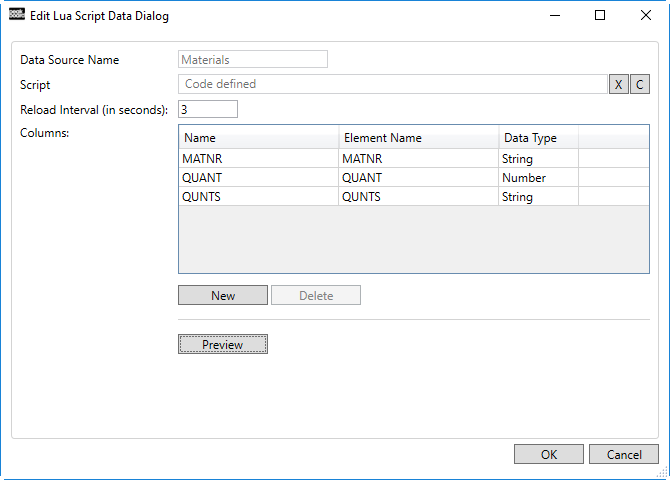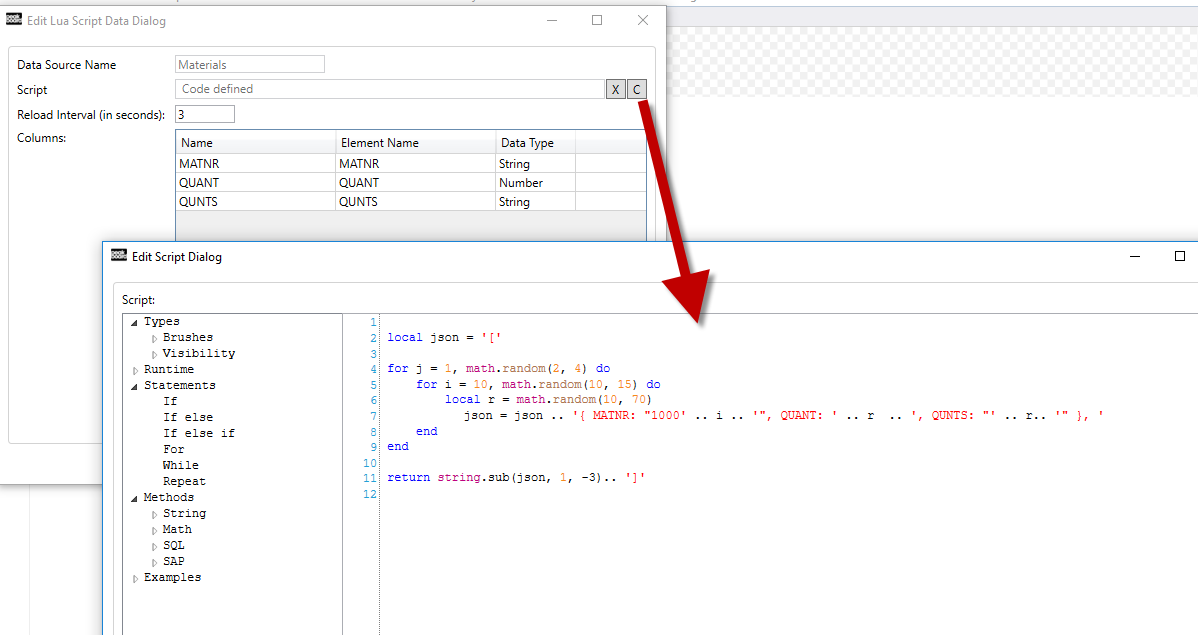# LUA脚本数据``````local json = '['

for j = 1, math.random(2, 4) do
for i = 10, math.random(10, 15) do
local r = math.random(10, 70)
json = json .. '{ MATNR: "1000' .. i .. '", QUANT: ' .. r .. ', QUNTS: "' .. r .. '" }, '
end
end

return string.sub(json, 1, -3).. ']'
``````